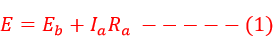Search
• Raj

# Torque Equation of DC Motor

Updated: Feb 27, 2021

The turning ability or capacity of the motor is known as torque. When the armature is fed DC supply the armature current starts flowing. If the armature current flows under the influence of magnetic flux, the armature conductor experience a force and the armature starts rotating. The DC motor diagram is as given below.Torque produced by DC Motor depends on the followings parameters.

1. Operational parameters - Magnetic flux and armature current

2. Design parameters - Number of poles P, Type of winding Lap or Wave, number of conductors(Z)

The EMF equation of the DC Motor is as follows.Multiplying both sides of equation(1), we getThe product of back EMF(Eb) and armature current(Ia) is the power developed by the motor.Back EMF of DC Motor can be expressed with the following mathematical expression.Putting the value of equation of back emf( eq.4) in equation(3)From equation( 5), it is clear that the torque produced by DC motor is proportional to the armature current(Ia) and the field flux.